# Abstract

When carrying out data analyses it is desirable to do so in a reproducible way. This aim is easier to achieve if a close link between the code, its documentation and the results is maintained. If done consistently this leads to reports that are relatively easy to maintain and can be updated automatically if either the data or details of the analysis change.

Here we are exploring the use of R package knitr and the document conversion tool pandoc to generate reproducible reports in R. After a general introduction to these two tools aspects relevant to the writing of scientific reports are discussed in some detail.

# Introduction

This is an introduction to the use of Markdown with embedded R code to create dynamic documents in multiple formats, e.g. HTML, PDF and Word. This is useful to generate reports (or papers) that contain all the relevant R code to carry out the analysis and allows for automatic updates to the document if either the code or the data change. As a result analyses become a lot easier to reproduce because the code and the presentation of results are closely linked and figures and tables can be updated automatically.

Traditionally dynamic R documents like this have been (and often still are) written in LaTeX using either Sweave or, more recently, knitr. While LaTeX is a very powerful tool that allows great control over page layout, the learning curve can be steep. More importantly, adding LaTeX commands to the text can be distracting and break the flow of writing and coding (at least for me) and the resulting LaTeX documents are not very readable. Of course they can be turned into beautiful PDFs but that doesn’t help while editing the text. More recently the use of Markdown has become popular. Writing Markdown is much easier than LaTeX, thus lowering the entry barrier, and its emphasis on maintaining readability of the raw text means that both writing and editing documents is faster than with LaTeX.

Several tools are available to produce dynamic documents in Markdown and convert them to various output formats. Here we will mainly focus on a combination of two of these, namely knitr and pandoc.

Much, if not all, of what is needed to create a reproducible analysis is provided by knitr. This R package provides functions that allow the processing of Markdown documents with embedded R code. The code will be executed and its output, including plots, can be included in the output. A selection of tutorials and useful examples for knitr can be found on knitr’s homepage. However, when trying to use this to generate publication quality reports the limitations of the Markdown syntax quickly start to become apparent. The focus on simplicity and the fact that it was originally designed for authoring web content means that much of the requirements for scientific writing are not easily met by standard Markdown.

Pandoc is a very useful tool that helps to alleviate this problem. It comes with its own Markdown dialect that includes many extensions that fill some of the gaps in the Markdown syntax, including the ability to use bibliographic databases in a variety of formats, while trying to retain the text’s readability. It also facilitates the conversion between a large number of document formats, providing great flexibility.

## Code conventions

Throughout this document examples of R code and Markdown formatting will be presented in code blocks:

message("This is R code")

To better show the effect of Markdown examples on the output these will often be followed by the same text rendered in the output format. To distinguish these examples from the main text the entire block of raw and converted Markdown will be framed by horizontal lines.

This is Markdown text in **bold** and *italics*.

This is Markdown text in bold and italics.

In addition to the code examples provided throughout this document the document itself is written in Markdown with embedded R code and may illustrate additional features.

## Availability

The HTML version of this document is available online. The PDF version is available for download and the source files are on GitHub.

## Compiling this document

Creating PDF and HTML output from the R/Markdown source file is a two step process. First knitr is used to execute the R code and produce the corresponding Markdown output. This can be done either by starting an R session and executing knitr("example.Rmd") or from the command line:

Rscript --slave -e "library(knitr);knit('example.Rmd')" 

Either way this generates a Markdown file called ‘example.md’. This can then be converted into PDF and HTML files by using the configuration file ‘example.pandoc’ by calling the pandoc function from the knitr package.

Rscript --slave -e "library(knitr);pandoc('example.md')"

The function automatically locates the configuration file and passes the requested parameters to pandoc.

### Required software

In addition to installations of knitr and pandoc a few external tools are required to compile this document.

R is required to run knitr as well as other R packages to support additional functionality.

• animation (for animated figures)
• pander (for Markdown formatting of R objects)

These can be installed via the install.packages command from within R. Animations also require ffmpeg and either ImageMagick or GraphicsMagick.

As one might expect a working LaTeX tool chain is required to generate PDF output from LaTeX documents. Several distributions are available online, including MiKTeX and TeX Live.

Python (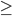2.7) is required for the pandoc filters discussed in the latter parts of this document. This also requires the pandocfilters Python module, which can be installed via pip.

# Brief Markdown primer

A Markdown formatted file is in essence a plain text file that may contain a number of formatting marks. It is designed to be easy to write and read in its raw form. Although it was originally designed as an easier way to write web pages it can be converted to many other rich text formats.

The purpose of this section is to briefly describe basic elements of Markdown formatting. More detailed descriptions are available online, e.g. at the official Markdown and pandoc websites.

The basics of text formatting involve marking of text as headings, structuring it into paragraphs and highlighting selected words for emphasis. Headings can be created by underlining them:

This is a top level heading
===========================
This is some ordinary text.

This is a second level heading
------------------------------
It is followed by more ordinary text.

Adding more "#" creates lower level headings

Note that pandoc also allows the use of “#” and “##” for first and second level headings.

Paragraphs are created by adding an empty line between two lines of text:

This is the first paragraph.
Line breaks are generally ignored in formatting.

This is the second paragraph.
If you add two or more spaces to the end of a line
the line break will be preserved in the conversion
to the output format.  

This is the first paragraph. Line breaks are generally ignored in formatting.

This is the second paragraph. If you add two or more spaces to the end of a line
the line break will be preserved in the conversion to the output format.

Several methods of highlighting text are supported:

Words within a paragraph and be *emphasised*. These are usually rendered in *italics*.
**Strong emphasis** typically results in **bold** text. Instead of * it is also possible
to use _ for emphasis. With pandoc it is also possible to ~~strike out~~ text.

Words within a paragraph and be emphasised. These are usually rendered in italics. Strong emphasis typically results in bold text. Instead of * it is also possible to use _ for emphasis. With pandoc it is also possible to strike out text.

## Block elements

### Block quotes

In Markdown quotes can be marked using the same conventions commonly used in email:

 > This text is quoted. A single ">" at the beginning
> of the paragraph is sufficient for the entire paragraph
> to be quoted (but syntax highlighting may not work properly).
>
> > You can also quote other quotes, i.e. block quotes can be nested.

This text is quoted. A single “>” at the beginning of the paragraph is sufficient for the entire paragraph to be quoted (but syntax highlighting may not work properly).

You can also quote other quotes, i.e. block quotes can be nested.

### Lists

Basic bullet lists can be created by starting a line with a *:

 * first item
* second item
* third item
• first item
• second item
• third item

1. first item
2. second item
3. third item
1. first item
2. second item
3. third item

but pandoc also allows this:

#. first item
#. second item
#. third item
1. first item
2. second item
3. third item

There is support for other of list types and variations of the basic syntax in pandoc. See the documentation for more details.

### Tables

Basic Markdown tables are created by lining up the columns and making headers, like so:

 Column 1    Column 2    Column 3
--------    --------    --------
1            10         100
2            20         200
3            30         300

Table: A simple table 
A simple table
Column 1 Column 2 Column 3
1 10 100
2 20 200
3 30 300

Just as with lists there are several variations and extensions to this basic syntax supported by pandoc. As usual, details can be found in the documentation.

### Code blocks and inline code

Special blocks to display source code with syntax highlighting can be included by starting a line with three back ticks, optionally followed by attributes to control aspects of the highlighting. A block like the one below will be rendered as R code.

r
x <- seq(-6,6, by=0.1)
yNorm <- dnorm(x)
yt <- dt(x, df=3)
yCauchy <- dcauchy(x)
plot(x, yNorm, type="l", ylab="Density")
lines(x, yt, col=2)
lines(x, yCauchy, col=4)
legend("topright", legend=c("standard normal", "t (df=2)", "Cauchy"),
col=c(1,2,4), lty=1)

x <- seq(-6,6, by=0.1)
yNorm <- dnorm(x)
yt <- dt(x, df=3)
yCauchy <- dcauchy(x)
plot(x, yNorm, type="l", ylab="Density")
lines(x, yt, col=2)
lines(x, yCauchy, col=4)
legend("topright", legend=c("standard normal", "t (df=2)", "Cauchy"),
col=c(1,2,4), lty=1)

Code fragments can also be included inline:

This is normal text with some R code: x <- runif(100){.r}.

This is normal text with some R code: x <- runif(100).

# Using knitr for dynamic code blocks

The code blocks we have seen so far are all static, i.e. while they do include valid source code this code is not interpreted, just displayed. To achieve the aim of a dynamic document that can be updated automatically if the underlying data or analysis change we need code blocks that are actually executed. The knitr R package does just that. Lets look again at the R code from the example in the previous section but this time we will use a code block that knitr will process.

    {r distributions}
x <- seq(-6, 6, by = 0.1)
yNorm <- dnorm(x)
yt <- dt(x, df = 3)
yCauchy <- dcauchy(x)


In this example syntac highlighting for the R code has been switched off to better demonstrate how the code chunks are created. Once the code in the above block has been evaluated we can use it for inline R statements that will be replaced with the computed values. For example we can do something like this:

The Normal density was evaluated at r length(yNorm) points.

The Normal density was evaluated at 121 points.

## Figures

To add a figure with a plot of the data all that is needed is to create the plot in an R chunk.

plot(x, yNorm, type = "l", ylab = "Density")
lines(x, yt, col = 2)
lines(x, yCauchy, col = 4)
legend("topright", legend = c("standard normal", "t (df=3)",
"Cauchy"), col = c(1, 2, 4), lty = 1)

This automatically includes the plot that was generated as a figure in the final document. It is possible to include a custom caption using the chunk option fig.cap.

## Animations

It is possible to include animations (generated from a series of plots) instead of a single figure. Look at this slightly more complex version of the previous example:

x <- seq(-6, 6, by = 0.1)
yNorm <- dnorm(x)
yCauchy <- dcauchy(x)
par(bg = "white")
for (i in 1:20) {
plot(x, yNorm, type = "l", ylab = "Density")
lines(x, dt(x, df = i), col = 2)
lines(x, yCauchy, col = 4)
legend("topright", legend = c("standard normal", paste0("t (df = ",
i, ")"), "Cauchy"), col = c(1, 2, 4), lty = 1)
}

It is possible to generate an animated GIF from this sequence of plots by wrapping the above code in a function and then calling saveGIF from the animation package.

threeDists <- function(df, x = seq(-6, 6, by = 0.1)) {
yNorm <- dnorm(x)
yCauchy <- dcauchy(x)
yt <- dt(x, df = df)
par(bg = "white")
plot(x, yNorm, type = "l", ylab = "Density")
lines(x, yt, col = 2)
lines(x, yCauchy, col = 4)
legend("topright", legend = c("standard normal", paste0("t (df = ",
df, ")"), "Cauchy"), col = c(1, 2, 4), lty = 1)
}

plotFun <- function(df) {
threeDists(df)
animation::ani.pause()
}

animation::saveGIF(lapply(1:20, plotFun), interval = 0.5, movie.name = "dist3.gif")
## Executing:
## 'convert' -loop 0 -delay 50 Rplot1.png Rplot2.png
##     Rplot3.png Rplot4.png Rplot5.png Rplot6.png
##     Rplot7.png Rplot8.png Rplot9.png Rplot10.png
##     Rplot11.png Rplot12.png Rplot13.png Rplot14.png
##     Rplot15.png Rplot16.png Rplot17.png Rplot18.png
##     Rplot19.png Rplot20.png 'dist3.gif'
## Output at: dist3.gif

The code above assumes that ImageMagick is installed. If you are using GraphicsMagick instead add the option convert="gm convert" to the saveGIF call.

Since the graphics output of this code is written directly to a file rather than an on-screen graphics device it will not be automatically included in the Markdown document produced by knitr. It can be included manually using the Markdown syntax for the inclusion of figures.

![Animated GIF of three related distributions](dist3) 

Note that this only works for output document formats that support GIFs. As a fallback we generate a png of the first frame to be included in other formats, e.g. PDF, that can’t display GIFs.

png("dist3.png")
threeDists(1)
dev.off()

Here we make use of pandoc’s --default-image-extension option to set the default image format to gif for HTML and docx output and to png for PDF.

### Creating animations on Windows

The procedure for generating animations may fail on Windows1. This may be due to a conflict between ImageMagick’s convert.exe, which is used to convert from png to gif, and Windows’ own convert.exe, which converts between FAT and NTFS file systems. It may be possible to circumvent this issue by using GraphicsMagick for the conversion instead. To do this, install GraphicsMagick and replace the call to saveGIF above with

animation::saveGIF(lapply(1:20, plotFun), interval=0.5, movie.name="dist3.gif",
convert="gm convert")

An alternative work-around involves bypassing the animation package entirely. Instead, rename ImageMagick’s convert.exe to imConvert.exe2, generate one png file for each frame of the animation and then call imConvert manually to create the animated gif.

png(file="figure/threeDist%02d.png", width=500, heigh=500)
lapply(1:20, threeDists)
dev.off()

shell("imConvert -delay 40 figure/threeDist*.png dist3.gif")

## Tables

It is often convenient to display the contents of R objects in the final document. While it is easy to simply display the output of R’s print statement as it would be displayed in the R console, this is not exactly producing pretty results. It is much more elegant to include proper tables that can be rendered nicely in the final document. Manually formatting the output as a Markdown table (that pandoc will then convert to the final output format) can be a daunting task. Fortunately R functions exist to help with this task. The knitr package provides a simple function, kable, that allows automatic formatting of tables. This requires the data to be in a suitable format (either a data.frame or a matrix) so some preprocessing may be necessary.

Consider the iris dataset distributed with R.

data(iris)
knitr::kable(head(iris))
Sepal.Length Sepal.Width Petal.Length Petal.Width Species
5.1 3.5 1.4 0.2 setosa
4.9 3.0 1.4 0.2 setosa
4.7 3.2 1.3 0.2 setosa
4.6 3.1 1.5 0.2 setosa
5.0 3.6 1.4 0.2 setosa
5.4 3.9 1.7 0.4 setosa

A table of summary statistics can be obtained with a little extra effort:

irisSummary <- apply(iris[, 1:4], 2, function(x) tapply(x, iris$Species, summary)) irisSummary <- lapply(irisSummary, do.call, what = rbind) This produces a list of matrices with summary statistics by iris species: irisSummary ##$Sepal.Length
##            Min. 1st Qu. Median Mean 3rd Qu. Max.
## setosa      4.3    4.80    5.0 5.01     5.2  5.8
## versicolor  4.9    5.60    5.9 5.94     6.3  7.0
## virginica   4.9    6.22    6.5 6.59     6.9  7.9
##
## $Sepal.Width ## Min. 1st Qu. Median Mean 3rd Qu. Max. ## setosa 2.3 3.20 3.4 3.43 3.68 4.4 ## versicolor 2.0 2.52 2.8 2.77 3.00 3.4 ## virginica 2.2 2.80 3.0 2.97 3.18 3.8 ## ##$Petal.Length
##            Min. 1st Qu. Median Mean 3rd Qu. Max.
## setosa      1.0     1.4   1.50 1.46    1.58  1.9
## versicolor  3.0     4.0   4.35 4.26    4.60  5.1
## virginica   4.5     5.1   5.55 5.55    5.88  6.9
##
## $Petal.Width ## Min. 1st Qu. Median Mean 3rd Qu. Max. ## setosa 0.1 0.2 0.2 0.246 0.3 0.6 ## versicolor 1.0 1.2 1.3 1.330 1.5 1.8 ## virginica 1.4 1.8 2.0 2.030 2.3 2.5 Each of these can again be displayed as a nicely formatted table using kable but unfortunately information about the column that was summarised will be lost in the process. For some output formats kable supports the use of a caption option but unfortuantely this doesn’t work when producing Markdown, as is the case here. An alternative is to use the pander package to produce output suitable for further processing with pandoc. suppressPackageStartupMessages(library(pander)) panderOptions("table.split.table", Inf) ## don't split tables pander(irisSummary[1:2]) • Sepal.Length: Min. 1st Qu. Median Mean 3rd Qu. Max. setosa 4.3 4.8 5 5.01 5.2 5.8 versicolor 4.9 5.6 5.9 5.94 6.3 7 virginica 4.9 6.22 6.5 6.59 6.9 7.9 • Sepal.Width: Min. 1st Qu. Median Mean 3rd Qu. Max. setosa 2.3 3.2 3.4 3.43 3.68 4.4 versicolor 2 2.52 2.8 2.77 3 3.4 virginica 2.2 2.8 3 2.97 3.18 3.8 Alternatively the following code produces somewhat more elegant output at the expense of a few extra lines of code. for (i in 3:4) { set.caption(sub(".", " ", names(irisSummary)[i], fixed = TRUE)) pander(irisSummary[[i]]) } Petal Length Min. 1st Qu. Median Mean 3rd Qu. Max. setosa 1 1.4 1.5 1.46 1.58 1.9 versicolor 3 4 4.35 4.26 4.6 5.1 virginica 4.5 5.1 5.55 5.55 5.88 6.9 Petal Width Min. 1st Qu. Median Mean 3rd Qu. Max. setosa 0.1 0.2 0.2 0.246 0.3 0.6 versicolor 1 1.2 1.3 1.33 1.5 1.8 virginica 1.4 1.8 2 2.03 2.3 2.5 The functionality provided by pander is alot more poweful than the simple kable function and can handle a wide variety of R objects. pander(t.test(Sepal.Length ~ Species == "setosa", data = iris)) Welch Two Sample t-test: Sepal.Length by Species == "setosa" Test statistic df P value Alternative hypothesis 15.14 147.4 7.709e-32 * * * two.sided # Converting from Markdown to multiple output formats using knitr Once R code chunks have been executed via knitr the resulting Markdown document can be converted to a variety of other formats with the help of pandoc. This generally works well but can require the construction of lengthy command lines. To make things worse these command lines may differ depending on the desired output format. If more than one output format is desired this can quickly become tedious. Fortunately knitr includes a function pandoc that takes care of the conversion process and can use a configuration file that lists all the desired options for the desired target formats. The configuration file used for this document is shown below3.  standalone: smart: normalize: toc: highlight-style: tango t: html5 self-contained: webtex: template: include/report.html5 c: include/buttondown.css default-image-extension: gif filter: include/equation.py include-in-header: include/equation.js include/affiliation.js t: latex latex-engine: xelatex template: include/report.latex V: geometry:margin=2cm geometry:driver=xetex documentclass:report classoption:a4paper H: include/captions.tex filter: include/equation.py default-image-extension: png t: docx default-image-extension: gif This file contains one block with format specific options for each output format, starting with t: <format>. Note that the first block has no target format specification and contains options that apply to all output formats. The use of a configuration file like this makes it easy to manage the (potentially large) number of options required to achieve the desired output. # Preparing manuscripts for publication Once an analysis has been completed and documented using techniques like the ones described above it may be desirable to use it as part of a publication without having to re-write it all. The purpose of this chapter is to investigate how well the authoring of scientific papers in Markdown is supported by the combination of knitr and pandoc and to demonstrate customisations to the default set-up where rquired. ## Requirements To be able to produce manuscripts that are suitable for submission to scientific journal several features are required. The purpose of this chapter is to explore to what extend the combination of knitr and pandoc can deliver a publication ready manuscripts and discuss simple extensions to add or enhance required features. Features essential to for a manuscript intended for submission to a journal are • References need to be cited throughout the text and listed at the end in a format specified by the journal. • Figures and tables need to be numbered and cross-references to these should be generated automatically, i.e. the numbers referred to in the text are updated automatically if the order of figures or tables changes. • Equations need to be rendered appropriately, numbered and cross-referenced where required. • A list of author names and affiliations needs to be displayed as part of the title block. • It has to be possible to preceed the main text with an abstract that may have to be formatted differently from the body of the manuscript. • Support for footnotes. ## Document meta information Documents may contain metadata blocks in YAML format. These blocks begin with three dashes --- and end with either three dashes --- or thee dots .... More than one metadata block can be present in the same document in which case conflicts caused by duplicate fields will be resolved by retaining the field that occurred first. Below is the metadata block used for this document.  --- title: Using knitr and pandoc to create reproducible scientific reports author: - name: Peter Humburg affiliation: 1 address: - code: 1 address: Wellcome Trust Centre for Human Genetics, University of Oxford, Roosevelt Dr., Oxford, OX3 7BN, UK date: Wed 15 Oct 2014 abstract: | When carrying out data analyses it is desirable to do so in a reproducible way. This aim is easier to achieve if a close link between the code, its documentation and the results is maintained. If done consistently this leads to reports that are relatively easy to maintain and can be updated automatically if either the data or details of the analysis change. Here we are exploring the use of R package knitr and the document conversion tool pandoc to generate reproducible reports in R. After a general introduction to these two tools aspects relevant to the writing of scientific reports are discussed in some detail. ...  Information gathered from metadata blocks is used by pandoc to populate metadata fields in the output document. This can be used to set the title, list of authors and abstract. Entries may contain (nested) lists and objects but note that the default templates make assumptions about the structure of specific fields. The author field in particular is expected to be a simple list or string. For the purpose of preparing reports or publications it may be convenient to use a richer structure, e.g. a list containing objects for name, affiliation and contact details. See the chapter on custom templates for details on how this structured author information might be used. ## Adding a bibliography Fortunately adding a list of refernces as well as citing them throughout the document is well supported by pandoc. References need to be contained in a bibliography file, which can be in a variety of formats (check the pandoc documentation for a list of supported formats). This file needs to be listed in the biblography entry of the document’s meta information. A bibliography consisting of all references that have been cited throughout the document will be generated by pandoc and added to the end of the document. The bibliography is formatted according to a format specified in a CSL style file. A browsable repository with a large number of different styles is available at http://zotero.org/styles. A citation is inserted into the text by adding the corresponding key (consisting of a ‘@’ followed by the citation’s identifier from the database) within square brackets. For example, [@smith04] would add a citation to the article with ID smith04 to the text and ensure that the corresponding bibliographic information is listed in the bibliography. Several variations of this are supported by pandoc, see the documentation for details. ## Better figure and table captions We already discussed how to generate figures and tables in knitr and we have seen that it is easy to add captions to these. However, so far all the figure and table cations were plain captions without any numbering. What we would like are figure captions that start with “Figure”, or “Fig.”, folowed by a number and a colon. There currently is no pandoc mechanism that allows to generate such captions in multiple output formats but there is an active and ongoing discussion that may lead to support for this in a future pandoc version. In the meantime we can use R to generate suitable labels when processing the input document with knitr. The following R function allows us to keep track of figures throughout the document, create appropriately numbered captions as well as cross-references:  1 2 3 4 5 6 7 8 9 10 11 12 13 14 15 16 17 18 19 20 21 22 23 24 25 26  figRef <- local({ tag <- numeric() created <- logical() used <- logical() function(label, caption, prefix = options("figcap.prefix"), sep = options("figcap.sep"), prefix.highlight = options("figcap.prefix.highlight")) { i <- which(names(tag) == label) if (length(i) == 0) { i <- length(tag) + 1 tag <<- c(tag, i) names(tag)[length(tag)] <<- label used <<- c(used, FALSE) names(used)[length(used)] <<- label created <<- c(created, FALSE) names(created)[length(created)] <<- label } if (!missing(caption)) { created[label] <<- TRUE paste0(prefix.highlight, prefix, " ", i, sep, prefix.highlight, " ", caption) } else { used[label] <<- TRUE paste(prefix, tag[label]) } } }) To get properly numbered figure captions all arguments to knitr‘s fig.cap chunk option have to be wrapped in a call to figRef with two arguments. The first argument is the label that should be used to refer to the figure and the second argument is the actual figure caption. The function is designed to allow some customisation. The prefix, e.g. ’Figure’ or ‘Fig.’, can be set via the prefix argument and the separator to be used between the number and the caption is set by the sep argument. It is also possible to adjust the formatting of the prefix in the figure caption, e.g. to display it with strong emphasis. For convenience the desired values can be stored together with other R options. Here we are setting the defaults to produce captions of the form “Figure N: caption text”. options(figcap.prefix = "Figure", figcap.sep = ":", figcap.prefix.highlight = "**") Calling this function with the label as its sole argument will create a reference while a call with two arguments (label and caption text) will create the actual figure caption. Consider the following example: {r carDataPlot, fig.cap=figRef("carData", "Car speed and stopping distances from the 1920s.")} plot(cars, xlab = "Speed (mph)", ylab = "Stopping distance (ft)", las = 1) lines(lowess(cars$speed, cars$dist, f = 2/3, iter = 3), col = "red")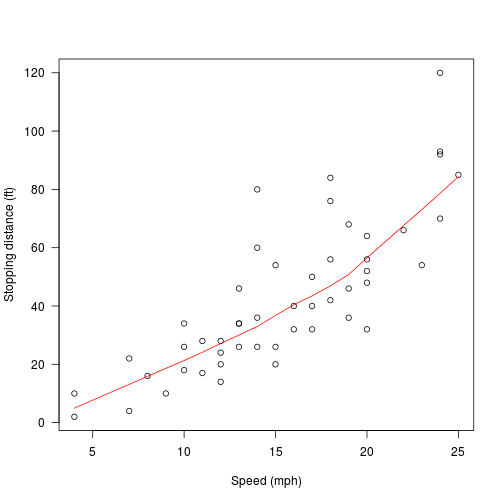Figure 1: Car speed and stopping distances from the 1920s. Now it is possible to refer back to this figure in the text using r figRef("carData"): Figure 1 shows a plot of car speeds and corresponding stop distances measured in the 1920s. Note the apparent non-linearity in the data. The log-scale data shown in Figure 2 has a more linear appearance. plot(cars, xlab = "Speed (mph)", ylab = "Stopping distance (ft)", las = 1, log = "xy") lines(lowess(cars$speed, cars$dist, f = 2/3, iter = 3), col = "red")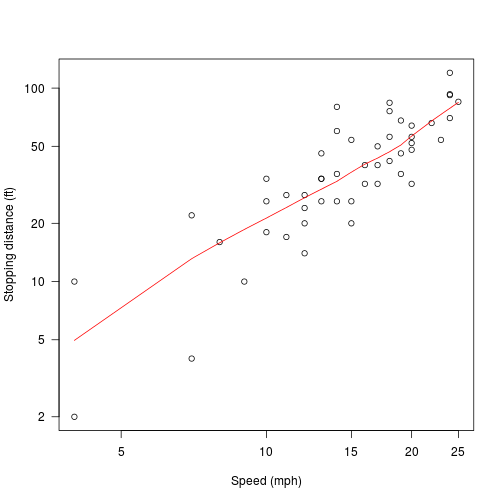Figure 2: Car speed and stopping distances on logarithmic scales. Note how this allows to refer to figures before they are are created. Although forward references should generally be avoided this isn’t always possible when it comes to figures. To ensure that all figures mentioned in the text actually exist the following code can be added to a knitr chunk at the end of the document. if (!all(environment(figRef)$created)) {
missingFig <- which(!environment(figRef)$created) warning("Figure(s) ", paste(missingFig, sep = ", "), " with label(s) '", paste(names(environment(figRef)$created)[missingFig],
sep = "', '"), "' are referenced in the text but have never been created.")
}
if (!all(environment(figRef)$used)) { missingRef <- which(!environment(figRef)$used)
warning("Figure(s) ", paste(missingRef, sep = ", "), " with label(s) '",
paste(names(environment(figRef)$used)[missingRef], sep = "', '"), "' are present in the document but are never referred to in the text.") } Figure 3 dosn’t exist and this generates a warning at the end of the document. The same approach can be used to obtain numbered table captions and corresponding references in the text.  1 2 3 4 5 6 7 8 9 10 11 12 13 14 15 16 17 18 19 20 21 22 23 24 25 26 27 28  tabRef <- local({ tag <- numeric() created <- logical() used <- logical() function(label, caption, prefix = options("tabcap.prefix"), sep = options("tabcap.sep"), prefix.highlight = options("tabcap.prefix.highlight")) { i <- which(names(tag) == label) if (length(i) == 0) { i <- length(tag) + 1 tag <<- c(tag, i) names(tag)[length(tag)] <<- label used <<- c(used, FALSE) names(used)[length(used)] <<- label created <<- c(created, FALSE) names(created)[length(created)] <<- label } if (!missing(caption)) { created[label] <<- TRUE paste0(prefix.highlight, prefix, " ", i, sep, prefix.highlight, " ", caption) } else { used[label] <<- TRUE paste(prefix, tag[label]) } } }) options(tabcap.prefix = "Table", tabcap.sep = ":", tabcap.prefix.highlight = "**") This can then be combined with the pander table generation technique demonstrated above. plot(cars, xlab = "Speed (mph)", ylab = "Stopping distance (ft)", las = 1, xlim = c(0, 25)) d <- seq(0, 25, length.out = 200) for (degree in 1:4) { fm <- lm(dist ~ poly(speed, degree), data = cars) assign(paste("cars", degree, sep = "."), fm) lines(d, predict(fm, data.frame(speed = d)), col = degree) } legend("topleft", legend = 1:4, col = 1:4, lty = 1)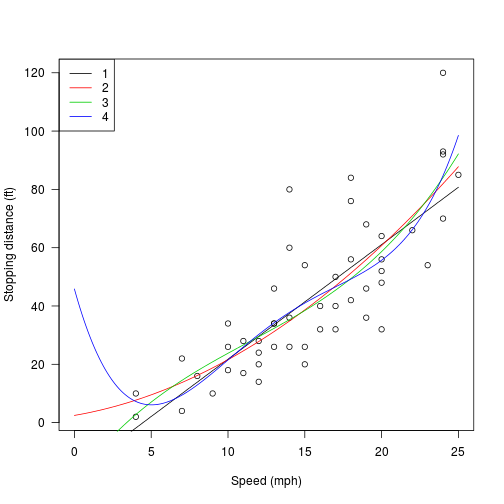Figure 4: Polynomial regression fits. set.caption(tabRef("carFit", "ANOVA table for polynomial regression fits to car speed and stopping distance data")) pander(anova(cars.1, cars.2, cars.3, cars.4)) Table 1: ANOVA table for polynomial regression fits to car speed and stopping distance data Res.Df RSS Df Sum of Sq F Pr(>F) 48 11354 NA NA NA NA 47 10825 1 528.8 2.311 0.1355 46 10634 1 190.4 0.8318 0.3666 45 10298 1 336.5 1.471 0.2316 This approach to figure and table captions has the advantage that it works for any output format and as such is well suited for situations where multiple output formats are required. The downside is that it doesn’t make use of any cross-referencing facilities that may be supported by one or several of the output formnats. For example, LaTeX has excellent support for this already and HTML output would benefit from the use of links to the actual figure or table. When a single output format is used it clearly makes sense to utilise the features it provides as much as possible. The multi-format approach presented here could be improved through the addition of some additional markup and a pandoc filter that turns this markup into format specific output. ## Structured author information By default pandoc only supports simple strings (or a list of strings) for the author field in the metadata block. This means that including information in addition to author names, e.g. affiliations and addresses, is dificult (but note that strings are interpreted as markdown so some formatting is possible). To really support the generation of publication ready documents the use of more structured author fields is desirable. For this document we use author information of the following form: author: - name: Author Name affiliation: 1 address: - code: 1 address: Department, Institution, Street address Other fields could be added to this, e.g. to indicate corresponding authors. In order for this additional information to be displayed in the output we need to extend the default templates to use this information. The required changes to the the HTML and LaTeX templates are discussed below. ## Equations There is good support for formula rendering in pandoc. Formulas can be written as TeX formulas between $ (for inline math) or $$ (for display math). These will be rendered in an output format specific way. In some formats, like HTML, the result depends on the command-line options used. This is a familiar bit of inline math: E=mc^2. This is a familiar bit of inline math: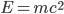. Here is an equation in display math mode:$$f(x, \mu, \sigma) = \frac{1}{\sigma\sqrt{2\pi}}e^{-\frac{(x-\mu)^2}{2\sigma^2}}$$ Here is an equation in display math mode: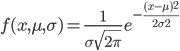While this generally works fairly well it doesn’t allow for numbered equations. A possible workaround is to use pandoc’s example list feature for this purpose. An axample list consists of consecutively numbered list elements that don’t have to be placed within the same list, i.e. they can be placed throughout the document. (@cauchy) f(x) = \frac{1}{\pi(1+x^2)} The Cauchy distribution (with density given in Eq. (@cauchy)) is a special case of Student's t-distribution (Eq. (@tdist)) with \nu = 1. (@tdist)$$f(t; \nu) = \frac{\Gamma(\frac{\nu+1}{2})} {\sqrt{\nu\pi}\,\Gamma(\frac{\nu}{2})} \left(1+\frac{t^2}{\nu} \right)^{-\frac{\nu+1}{2}}$$ 1.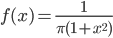The Cauchy distribution (with density given in Eq. (1)) is a special case of Student’s-distribution (Eq. (2)) with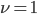. 1.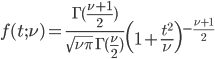While this solves the basic problem of getting numbered equations it isn’t perfect. Equations are not centred and numberes appear on the left rather than the right as is customary. An additional problem when using display math (as in Eq. (2)) is that the number and the equation are not lined up properly. It is possible to fix this in HTML output through the use of appropriate CSS but that doesn’t help for other output formats. <div class="equation"> (@gamma)$$\Gamma(t) = \int^\infty_0 x^{t-1}e^{-x}dx</div> 1.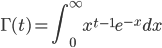In the above example the equation is wrapped in a div with class “equation”. This allows application of suitable CSS to improve the alignment of the equation. Horizontal alignment of the formula is relatively straightford with CSS so it can be centred without too much difficulty in HTML output. Proper alignment with the automatically generated number proves to be more difficult. The following javascript code does the trick.  1 2 3 4 5 6 7 8 9 10 11 12 13 14 15 16 17 18 19 20 21 22 23 24 25 26 27    This isn’t particularly elegant and only solves the problem for HTML. For LaTeX the lack of proper equation handling is particularly unsatisfying as LaTeX has much better support for equations. Again using a filter for additional processing to produce equations that are better suited to the output format. This should allow the use of LaTeX equation environments in LaTeX output and could be used to produce better HTML output as well. This filter can also make use of the div introduced above. See below for an example of how this can be achieved. ## Footnotes The use of footnotes is well supported by pandoc. The easiest way to add a footnote is the inline syntax. This is regular text^[with a footnote]. This is regular text4. It is also possible to use labels to identify a footnote, similar to the way references work. When using the reference style a short label is present in the text[^1] and the actual footnote text is defined elsewhere. [^1]: This is closer to the appearance of the rendered text in the output but updating the footnote text is a little bit more work since it may be somewhere else in the document. The advantage of this format is that it supports multi-block content. It could even contain a code block if desired. r message("This is R code")  The trick is to indent subsequent paragraphs to indicate that they form part of the footnote. Afterwards the normal text continues. When using the reference style a short label is present in the text5 and the actual footnote text is defined elsewhere. Afterwards the normal text continues. # Customising output Pandoc provides default templates for all supported output formats. These provide a quick and easy way to generate output in a variety of formats. Typically they produce decent looking results and in some cases will be all that is required. However, complex documents for large projects often benefit from some customisation. ## Using custom headers Many output formats use header information to specify details of the output rendering. Modifying the header can give substantial control over the appearance of the final output. Using the -H option of pandoc allows the contents of arbitrary files to be added to the header of a template. For example, to tweak the appearance of figure and table captions the following file is included for the LaTeX output of this document.  \usepackage[format=hang,labelformat=empty,labelsep=none]{caption} Multiple files with header content can be included in this way, allowing for a some flexibility and the option to create re-usable header fragments. ## Custom templates Sometimes more substantials modifications are called for. In this case it may be possible to create a custom template that incorporates the desired changes. The best way to create a custom template is to start with the default template for the desired output format and modify it as necessary. The latest version of all pandoc templates are available on GitHub. While it is possible to simply download the desired file and modify it, the recommended way of producing custom templates is to fork the repository and then commit all changes to the forked version. This makes it easier to update the customised version of the template with changes to the default6. This may be necessary to accomodate changes to pandoc. Templates allow access to pandoc’s template variables. All variable expressions are surrounded by : variable. These can be used in conditionals, adjusting the contents of the output accordingly. if(variable) variable else Some default text. endif In cases where a variable contains a list it is possible to iterate over its elements, inserting each into the text. For exxample, the default latex template has the following code to populate the author field. if(author) \author{for(author)authorsep \and endfor} endif Note that sep allows the specification of a separator to be used between list elements. ### Including an abstract in HTML output Customising templates can be particularly useful when the default doesn’t handle certain types of metadata that should be displayed in the output. For example, this document contains an abstract that is included in the PDF output but ignored in the HTML output by default. This can be changed by adding a few lines to the HTML5 template. if(abstract) <section class="abstract"> <h1 class="abstract">Abstract</h1> abstract </section> endif Adding this between the header block and the table of contents block has the desired effect of including the text of the abstract near the top of the page. This can then be styled with CSS as desired. The style sheet used here has the following: h1.abstract { text-align: center; } section.abstract { width:50%; margin-left:auto; margin-right:auto; background:#eef; padding:10px; } ### Using structured author fields LaTeX, unsurprisingly, has pretty good support for additional author information. Here we use the authblk package to typeset the addresses. This allows us to specify authors and their affiliations through a shared identifier. This conveniently matches the format used in the metadata block. Here is the (somewhat more complex) template code to insert author information with optional affiliation.  1 2 3 4 5 6 7 8 9 10 11 12 13 14 15 16 17 18 19  if(author) \usepackage{authblk} if(address) for(author) \author[author.affiliation]{author.name} endfor for(address) \affil[address.code]{address.address} endfor else for(author) if(author.name) \author{author.name} else \author{author} endif endfor endif endif This supports multiple authors and can handle both the new structured author blocks as well as the original plain author field. However, in its current for it only supports a single affiliation per author. Note how line 5 uses the author.affiliation field: \author[author.affiliation]{author.name}. This assumes a single string but can be modified to handle a list of strings instead by wrapping the variable in a for loop: \author[for(author.affiliation)author.affiliationsep, endfor]{author.name}. This largely works as intended with the only limitation that the IDs used to associate authors with intitutions are directly used as superscripts to the author’s name. It would be desirable to generate a sequence of consecutive numbers (or other LaTeX symbols) automatically but that would require further processing. As one might expect direct support for structured author information in HTML output is a bit more limited. The modification to the template follow similar lines.  1 2 3 4 5 6 7 8 9 10 11 12 13 14 15 16 17 18 19 20 21 22 23 24 25  if(author) if(address) Author Affiliations endif endif The addresses are added as an ordered list (lines 17 - 25), providing us with automatic numbering. The corresponding IDs are stored in a data attribute of the list elements as well as with the author names. To generate the matching superscripts to the names we use a few lines of javascript.  1 2 3 4 5 6 7 8 9 10 11 12 13 14 15 16 17 18 19 20 21 22 23 24 25 26 27 28 29    Unlike the LaTeX solution this supports the use of arbitrary IDs but requires some extra processing of the output. It also relies on javascript being enabled in the browser. The appearance of the address information can then be modified with CSS. Here we also use additional javascript to toggle the display when the heading is clicked. function toggleDisplay(d) { if(document.getElementById(d).style.display == "none") { document.getElementById(d).style.display = "block"; } else { document.getElementById(d).style.display = "none"; } } # Pandoc filters It is possible to add additional processing steps to format conversions in pandoc through the use of filters. A filter is a small (or possibly rather complex) program that is executed by pandoc after the contents of the input file has been transformed into pandoc’s native (JSON) representation. The filter can then modify that representation as desired and the resulting document is then converted to the target format. The filter has access to the requested target format and can therefore be used to make format specific modifications. This can, e.g., be used to wrap equations in appropriate environments in LaTeX. Since pandoc is written in Haskell this is also the language best suited to writing filters. However, there is a Python module (pandocfilters) providing support for the parsing and writing of pandoc’s native format. Once a filter has been written, and assuming that it is available in an executable form, it can be passed to pandoc through the --filter command-line option. ## Better equations For the remainder of this chapter we will discuss a filter designed to provide better equations in LaTeX and HTML output. The aim is to use the equation environment in LaTeX for individual, numbered equations and the align environment for groups of equations that should be lined up with each other. In HTML output these should be rendered in a similar way, i.e. equations are centred with numbering on the right and equations are lined up correctly where applicable. Here are a few equations we will use for testing: <div id="volterra" class="equation"> (@volterra)\frac{dx}{dt} = x(\alpha - \beta y)\frac{dy}{dt} = - y(\gamma - \delta  x)$$</div> The system of ordinary differential equations given by Eq. <span id="volterra" class="eq_ref">(@volterra)</span> is commonly used to describe predator-prey systems. Any solution to this system of equations satisfies the equality in Eq. <span id="volterra_constant" class="eq_ref">(@volterra_constant)</span>. <div id="volterra_constant" class="equation"> (@volterra_constant)$$V = -\delta \, x + \gamma \, \log(x) - \beta \, y + \alpha \, \log(y)
</div>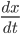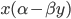(1)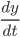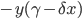(2)

The system of ordinary differential equations given by Eq. (1) is commonly used to describe predator-prey systems. Any solution to this system of equations satisfies the equality in Eq. (3).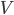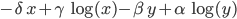(3)

### Python filter implementation

This section provides a step-by-step explanation of the filter implementation. The full code of the final script is available in the appendix. The Python script for achieving the desired equation formatting needs to import the pandocfilters module. We will also need regular expressions.

#! /usr/bin/env python

from pandocfilters import toJSONFilter, RawBlock, Div
import re

The pandocfilters module provides the function toJSONFilter. This function takes another function as its sole argument and applies it to each node of the JSON document that is read from standard input. To be able to pass this script to pandoc as one of the command-line options it needs to include the following two lines.

if __name__ == '__main__':
toJSONFilter(equation)

Of course we also need to define the function equation. This function has to accept four arguments corresponding to the key and value of the current node, the requested target format and the document’s meta information.

def equation(key, value, format, meta):
# process equation nodes

Whenever this function returns a value this value will replace the current node. If nothing is returned the current node is unchanged. The return value can be a single object or a list of objects. To this end we will need to generate Objects representing raw LaTeX and HTML code to add the desired formatting. To this end we define the following two functions with the help of element constructors provided by pandocfilters.

def latex(x):
return RawBlock('latex',x)

def html(x):
return RawBlock('html', x)

The key is a short string identifying the type of node that is currently being processed. Since we have chosen to wrap all equations in a div with class ‘equation’ we need to check this and proceed with processing whenever one of these divs is encountered.

  if key == 'Div':
[[ident,classes,kvs], contents] = value
if 'equation' in classes:
# process equation

Once we have identified the div we need to extract the actaul equations from the contents. Note that this may contain either one or several equations and these may be wrapped in an ordered list (if the example list style numbering system is used for other output formats). The following function extracts the contents of math environments.

def getMath(x):
if isinstance(x, list):
return [getMath(l) for l in x]
if isinstance(x, dict):
if x['t'] == 'Math':
return x['c']
else:
return getMath(x['c'])

We need to apply this to all sub-nodes of the div. To facilitate the traversal of the corresponding nested list the function below is used.

def iter_flatten(iterable):
it = iter(iterable)
for e in it:
if isinstance(e, (list, tuple)):
for f in iter_flatten(e):
yield f
else:
yield e

Together these functions allow us to generate a list of equations:

math = iter_flatten([ getMath(contents)])
math = [x for x in math if x is not None]

Note that this produces a list of lists with elements that may be empty or None (because not all nodes contain equations). The list comprehension in the second line above is used to flatten the list and remove all unwanted entries.

### LaTeX output

With the actual equations extracted from the div the main task remaining is to format them appropriately for the desired output format. If the div contains a single equation we’ll use the equation environment for LaTeX output. If there are multiple equations grouped to gether the align environment is used to create a nicely lined-up block of equations. The align environment in LaTeX uses & to define alignment points. We want to allow manual alignment of equations, meaning that existing & symbols need to be respected. In the absence of a predefined alignment we’ll align equations on the first relational operator7. This is achieved with the function below.

def alignLatexMath(x):
global relSymbol
relPattern = '|'.join(relSymbol)
if re.search(r'[^\\]&', x) is None:
idx = re.search(relPattern, x).start()
return x[:idx] + '&' + x[idx:]
return x

The following code fragment then generates the LaTeX output.

if format == 'latex':
type = 'equation'
if len(math) > 1:
type = 'align'
math = [alignLatexMath(x) for x in math]
if ident != '':
label = '\\label{' + ident + '}'
else:
type = type + '*'
return [latex('\\begin{' + type + '}' + label + "\n" + \
"\\\\\n".join(math) + \
"\n" + '\\end{' + type + '}')]

Note that this adds a label to the environment based on the ID of the div (if present) and for equations without label the numbering is suppressed. In cases where the align environment is used only the first equation will be labelled with the provided ID and all subsequent equations will be assigned labels of the form ID.n, starting with n = 1 and increasing by one for each subsequent equation. While this allows referencing of individual equations within an align environment it can be difficult to maintain these references if new equations get inserted into the middle of a block.

### HTML output

For HTML output we’ll use a table with appropriate CSS styling to obtain a similar effect. Since we can’t rely on LaTeX’s build-in facilities for handeling equations this requires slightly more effort.

The output consists of three columns per equation. These are used for the left and right sides of the equation with the relational operator in the middle. If multiple columns of equations are required these three columns will be repeated for each. If the div is labelled an additional column is added at the end to contain the equation number8.

To prepare equations for formatting we split them at the alignment point.

def alignHtmlMath(x):
global relSymbol
relPattern = r'|'.join(relSymbol)
cols = re.split(r"[^\\]&(?!" + relPattern + ")", x)
align = [re.search(r"[^\\]&", x) for x in cols]
out = []
for i in range(len(align)):
if align[i] is not None:
idx = align[i].start()
skip = 1
else:
idx = re.search(relPattern, cols[i]).start()
skip = 0
out = out + [[cols[i][:idx+skip], cols[i][idx+2*skip:idx+2*skip+1],
cols[i][idx+2*skip+1:]]]
return out

math = [alignHtmlMath(x) for x in math]

Now we only need to decorate the resulting equation fragments with appropriate HTML tags to create the table.

if ident != '':
label = 'id=\"' + ident + '\" '
head = [html('<table ' + label + 'class=\"' + ' '.join(classes) + '\" ' + \
' '.join(kvs) + '>' + "\n")]
tail = [html('</table>' + "\n")]
body = [html('<tbody>' + "\n")]
for eq in math:
eqCount = eqCount + 1
body = body + [html('<tr>' + "\n")]
for sub in [formatHtmlMath(y) for y in eq]:
body = body + sub
if ident != '':
body = body + [html(' <td class=\"eq_number\"> <br>(' + \
str(eqCount) + ')<br> </td>')]
body = body + [html('</tr>' + "\n")]
body = body + [html('</tbody>' + "\n")]

### Styling equations with CSS

Now that we have a filter that generates HTML output that is structured to allow proper layout of equations the only missing piece is the actual layout. A little bit of CSS should take care of that.

We start by setting the table to span the full width of the content div. This will allow us to centre the equation while displaying the equation number at the right margin.

table.equation
{
width: 100%;
}

Unfortunately this results in spreading the equation out across the entire line. To achieve the desired effect we define a nostretch style for table cells and apply it to the cells holding the central and right part of the equation.

td.nostretch
{
width: 1%;
}

The result of this is that the the left and rightmost cells will stretch to fit the width of the contents div. All that is left to do is to ensure that the cotents of these cells is aligned properly.

table.equation td:not(nostretch)
{
text-align: right;
}

table.equation td.eq_right
{
text-align: left;
}

We need an extra rule to ensure that the right part of the equation is aligned left so that it is flush with the central part even when there are multiple rows with different length of contents for this column.

### Keeping track of references

Now equations are properly displayed and numbered in both HTML and LaTeX output but unfortunately the cross-references in the text (generated via the example list mechanism) are no longer garuanteed to match the numbers of the actual equations. In HTML output the numbers should match if all equations contained in equation divs are numbered but LaTeX may use a different numbering scheme, e.g. numbering equations by chapter. Again it would make sense to utilise LaTeX’s inherent abilities (this time for cross-referencing) and to add some additional code to achieve the same effect with HTML. It would also be nice to retain the example list method for numbering equations as a fall back for other output formats.

To achieve this we will again resort to additional markup, in this case a span element: markdown <span id="label" class="eq_ref">(@label)</span>. This can the be processed by our equation filter by adding just a few extra lines of code.

if key == 'Span':
[[ident,classes,kvs], contents] = value
if 'eq_ref' in classes:
if format == 'latex':
return latexInline("(" + "\\ref{" + ident + "})")              

For convenience we have also introduced a new (very simple) function.

def latexInline(x):
return RawInline('latex', x)

For HTML output things are a bit more complicated as we have to keep track of equation numbers. We’ll use a dictionary to store labels and corresponding numbers for equations. Whenever a new equation label is encountered in the document it has to be added to the dictionary. The function eqNumber can be called with a label and will return the corresponding equation number (registering the label in the process if it hasn’t occoured before).

_eqLabel = {}
def eqNumber(id):
global _eqLabel
if id not in _eqLabel.keys():
_eqLabel[id] = len(_eqLabel) + 1
return str(_eqLabel[id])

# Appendix

## Equation filter

 1 2 3 4 5 6 7 8 9 10 11 12 13 14 15 16 17 18 19 20 21 22 23 24 25 26 27 28 29 30 31 32 33 34 35 36 37 38 39 40 41 42 43 44 45 46 47 48 49 50 51 52 53 54 55 56 57 58 59 60 61 62 63 64 65 66 67 68 69 70 71 72 73 74 75 76 77 78 79 80 81 82 83 84 85 86 87 88 89 90 91 92 93 94 95 96 97 98 99 100 101 102 103 104 105 106 107 108 109 110 111 112 113 114 115 116 117 118 119 120 121 122 123 124 125 126 127 128 129 130 131 132 133 134 135 136 137 138 139 140 141 142 143 144 145 146 147 148 149 150 151 152 153 154 155 156 157 158 159 160 161 162 163 164 165 166 167 168 169 170 171 172   #! /usr/bin/env python """ Pandoc filter to convert the contents of divs with class 'equation' to equation environments when producing latex output. If the div contains a single math block the equation environment is used and a label matching the ID of the div (if present) is placed inside. If the div contains more than one math block the align environment is used instead but only the first equation is labelled. Alignment characters present in the equations ('&') are honoured. Equations without predefined alignment are aligned on the first '='. """ from pandocfilters import toJSONFilter, RawBlock, RawInline import re import sys def latex(x): return RawBlock('latex',x) def latexInline(x): return RawInline('latex', x) def html(x): return RawBlock('html', x) def htmlInline(x): return RawInline('html', x) def DisplayMath(x): return { u'c': [{u'c': [{u'c': [], u't': u'DisplayMath'}, x], u't': u'Math'}], u't': u'Plain'} def iter_flatten(iterable): it = iter(iterable) for e in it: if isinstance(e, (list, tuple)): for f in iter_flatten(e): yield f else: yield e def getMath(x): if isinstance(x, list): return [getMath(l) for l in x] if isinstance(x, dict): if x['t'] == 'Math': return x['c'] else: return getMath(x['c']) def alignLatexMath(x): global relSymbol relPattern = '|'.join(relSymbol) if re.search(r'[^\\]&', x) is None: idx = re.search(relPattern, x).start() return x[:idx] + '&' + x[idx:] return x def alignHtmlMath(x): global relSymbol relPattern = r'|'.join(relSymbol) cols = re.split(r"[^\\]&(?!" + relPattern + ")", x) align = [re.search(r"[^\\]&", x) for x in cols] out = [] for i in range(len(align)): if align[i] is not None: idx = align[i].start() skip = 1 else: idx = re.search(relPattern, cols[i]).start() skip = 0 out = out + [[cols[i][:idx+skip], cols[i][idx+2*skip:idx+2*skip+1], cols[i][idx+2*skip+1:]]] return out def formatHtmlMath(eq, numbered=True): if numbered: finalStretch = ' nostretch' else: finalStretch = '' return [html('
')] + \ [DisplayMath(eq)] + \ [html(' ')] + \ [DisplayMath(eq)] + \ [html(' ')] + \ [DisplayMath(eq)] + \ [html('
' + "\n")] + \ [html(' ' + "\n")] + \ [html(' ' + "\n")] def eqNumber(id): global _eqLabel if id not in _eqLabel.keys(): _eqLabel[id] = len(_eqLabel) + 1 return str(_eqLabel[id]) def eqLabel(id): eqNumber(id) return id relSymbol = ['\\leq', '\\geq', '\\equiv', '\\models', '\\prec', '\\succ', '\\sim' '\\perp', '\\preceq', '\\succeq', '\\simeq', '\\mid', '\\ll', '\\gg', '\\asymp', '\\parallel', '\\subset', '\\supset', '\\approx', '\\bowtie', '\\subseteq', '\\supseteq', '\\cong', '\\Join', '\\sqsubset', '\\sqsupset', '\\neq', '\\smile', '\\sqsubseteq', '\\sqsupseteq', '\\doteq', '\\frown', '\\in', '\\ni', '\\propto', '=', '\\vdash', '\\dashv', '<', '>'] _eqLabel = {} def equation(key, value, format, meta): if key == 'Div': [[ident,classes,kvs], contents] = value if 'equation' in classes: math = iter_flatten([ getMath(contents)]) math = [x for x in math if x is not None] id = [''] * len(math) if ident != '': id = [eqLabel(ident)] if len(math) > 1: for i in xrange(1, len(math)): id = id + [eqLabel(ident + '.' + str(i))] if format == 'latex': type = 'equation' if len(math) > 1: type = 'align' math = [alignLatexMath(x) for x in math] if ident != '': label = ['\\label{' + id + '}' for id in id] else: type = type + '*' label = id math = zip(math, label) return [latex('\\begin{' + type + '}' + "\n" + \ "\\\\\n".join([e + ' ' + l for (e, l) in math]) + \ "\n" + '\\end{' + type + '}')] if format == 'html' or format == 'html5': math = [alignHtmlMath(x) for x in math] if ident != '': label = ['id=\"' + x + '\" ' for x in id] else: label = id head = [html('' + "\n")] tail = [html('' + "\n")] body = [html('

(' + eqNumber(id[i]) + ')
' + "\n")] for (i, eq) in enumerate(math): body = body + [html('' + "\n")] for sub in [formatHtmlMath(y, ident != '') for y in eq]: body = body + sub if ident != '': body = body + [html(' ')] body = body + [html('' + "\n")] body = body + [html('' + "\n")] return head + body + tail if key == 'Span': [[ident,classes,kvs], contents] = value if 'eq_ref' in classes: if format == 'latex': return latexInline("(" + "\\ref{" + ident + "})") if format == 'html' or format == 'html5': return htmlInline("(" + eqNumber(ident) + ")") if __name__ == '__main__': toJSONFilter(equation)

## Session info

R version 3.1.0 (2014-04-10) Platform: x86_64-pc-linux-gnu (64-bit)

locale:  LC_CTYPE=en_GB.UTF-8 LC_NUMERIC=C
 LC_TIME=en_GB.UTF-8 LC_COLLATE=en_GB.UTF-8
 LC_MONETARY=en_GB.UTF-8 LC_MESSAGES=en_GB.UTF-8
 LC_PAPER=en_GB.UTF-8 LC_NAME=C
 LC_MEASUREMENT=en_GB.UTF-8 LC_IDENTIFICATION=C

attached base packages:  stats graphics grDevices utils datasets  methods base

other attached packages:  pander_0.3.9 knitr_1.6

loaded via a namespace (and not attached):  animation_2.3 digest_0.6.4 evaluate_0.5.5  formatR_0.10 Rcpp_0.11.2 stringr_0.6.2  tools_3.1.0

## Warning: Figure(s) 3 with label(s) 'missingFigure' are referenced in the text but have never been created.
## Warning: Figure(s) 4 with label(s) 'carFit' are present in the document but are never referred to in the text.

1. Thanks to reyntjesr for reporting this issue and providing the work around described here.

2. Instead of renaming the file it is also possible to use the full path to ImageMagick’s convert.exe in the shell command.

3. This also demonstrates another feature of knitr: It is possible to include external documents using the child chunk option

4. with a footnote

5. This is closer to the appearance of the rendered text in the output but updating the footnote text is a little bit more work since it may be somewhere else in the document.

The advantage of this format is that it supports multi-block content. It could even contain a code block if desired.

{code.block}

The trick is to indent subsequent paragraphs to indicate that they form part of the footnote.

6. see https://help.github.com/articles/syncing-a-fork for a description of how to do this

7. The align environment allows the use of multiple alignment points to group equations into columns. Automatic alignment generated by this filter only supports a single alignment point, set at the first relational operator. If you want a more complex alignment all equations in a block should define the required alignment points.

8. Here we are using a global variable (eqCount) in the python script to keep track of the number of equations. We could use a CSS counter instead to insert the number into the web page as it is rendered in the browser. If all we wanted to do was to number the equations that would probabaly be the better solution but we will proceed to extend this to allow cross-references as well and CSS can’t really handle those.